Courses

# Olympiad Test: Simple Interest - 1

## 20 Questions MCQ Test Mathematics Olympiad Class 7 | Olympiad Test: Simple Interest - 1

Description
This mock test of Olympiad Test: Simple Interest - 1 for Class 7 helps you for every Class 7 entrance exam. This contains 20 Multiple Choice Questions for Class 7 Olympiad Test: Simple Interest - 1 (mcq) to study with solutions a complete question bank. The solved questions answers in this Olympiad Test: Simple Interest - 1 quiz give you a good mix of easy questions and tough questions. Class 7 students definitely take this Olympiad Test: Simple Interest - 1 exercise for a better result in the exam. You can find other Olympiad Test: Simple Interest - 1 extra questions, long questions & short questions for Class 7 on EduRev as well by searching above.
QUESTION: 1

### A person borrows Rs 5000 for 2 years at 4% p.a. simple interest. He immediately lends it to another person at 6.25% p.a. for 2 years. Find his gain in the transaction per year.

Solution:

Gain in 2 years
= Rs [(5000 × 25/4 × 2/100)
– {(5000 × 4 × 2)/100}]
= Rs (6 25 – 400)
= Rs 225
Gain in 1 year = Rs 225/2 =112.50

QUESTION: 2

### A certain amount earns simple interest of Rs 1750 after 7 years. Had the interest been 2% more, how much more interest would it have earned?

Solution:

We need to know the S.I., principal and time to find the rate.
Since the principal is not given, so data is inadequate.

QUESTION: 3

### What will be the ratio of simple interest earned by certain amount at the same rate of interest for 6 years and that for 9 years?

Solution:

Let the principal be P and rate of interest be R%.
Required ratio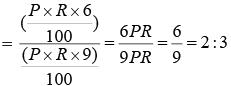QUESTION: 4

A sum of Rs 12,500 amounts to Rs 15,500 in 4 years at the rate of simple interest. What is the rate of interest?

Solution:

S.I. = Rs (15500 – 12500) = Rs 3000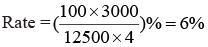QUESTION: 5

An automobile financier claims to be lending money at simple interest, but he includes the interest every six months for calculating the principal. If he is charging an interest of 10%, the effective rate of interest becomes:

Solution:

Let the sum be Rs 100. Then,
S.I. for first 6 months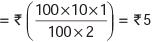S.I. for last 6 months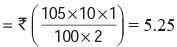So, amount at the end of 1 year
= Rs (100 + 5 + 5.25) = Rs 110.25
Effective rate = (110.25 – 100) = 10.25%

QUESTION: 6

A lent Rs 5000 to B for 2 years and Rs 3000 to C for 4 years on simple interest at the same rate of interest and received Rs 2200 in all from both of them as interest. The rate of interest per annum is:

Solution:

Let the rate be R% p.a.
Then,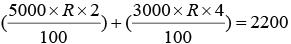⇒ 100R + 120R = 2200
⇒ R = 2200/220
Rate = 10%.

QUESTION: 7

A sum of Rs 725 is lent in the beginning of a year at a certain rate of interest. After 8 months, a sum of Rs 362.50 more is lent but at the rate twice the former. At the end of the year, Rs 33.50 is earned as interest from both the loans. What was the original rate of interest?

Solution:

Let the original rate be R%. Then, new rate = (2R) %.
Note: Here, original rate is for 1 year(s);
the new rate is for only 4 months i.e. 1/3 year(s).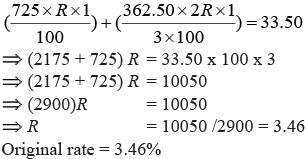QUESTION: 8

A man took loan from a bank at the rate of 12% p.a. simple interest. After 3 years he had to pay Rs 5400 interest only for the period. The principal amount borrowed by him was:

Solution:

Principal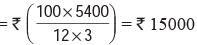QUESTION: 9

A sum of money amounts to Rs 9800 after 5 years and Rs 12005 after 8 years at the same rate of simple interest. The rate of interest per annum is:

Solution:

S.I. for 3 years = Rs (12005 – 9800)
= Rs 2205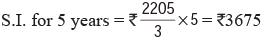Principal = Rs (9800 – 3675) = Rs 6125
Hence, rate = (100 × 3675)/(6125 × 5) = 12%

QUESTION: 10

A sum of money at simple interest amounts to Rs 815 in 3 years and to ` 854 in 4 years. The sum is:

Solution:

S.I. for 1 year = Rs (854 – 815) = Rs 39
S.I. for 3 years = Rs (39 × 3) = Rs 117
∴ Principal = Rs (815 – 117) = Rs 698

QUESTION: 11

Mr. Thomas invested an amount of Rs 13,900 divided in two different schemes A and B at the simple interest rate of 14% p.a. and 11% p.a. respectively. If the total amount of simple interest earned in 2 years be ` 3508, what was the amount invested in Scheme B?

Solution:

Let the sum invested in Scheme A be Rs x and that in Scheme B be Rs (13900 – x).
Then,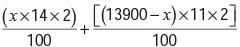= 3508
⇒ 28x – 22x = 350800 – (13900 × 22)
⇒ 6x = 45000
⇒ x = 7500
So, sum invested in Scheme B
= Rs (13900 – 7500) = Rs 6400

QUESTION: 12

A sum fetched a total simple interest of Rs 4016.25 at the rate of 9% p.a. in 5 years. What is the sum?

Solution:

Principal = Rs (100 × 4016.25)/(9 × 5)
= Rs 401625/45
= Rs 8925

QUESTION: 13

How much time will it take for an amount of Rs 450 to yield Rs 81 as interest at 4.5% per annum of simple interest?

Solution:

Time = (100 × 81)/ (450 × 4.5) years
= 4 years

QUESTION: 14

Reena took a loan of  Rs 1200 with simple interest for as many years as the rate of interest. If she paid Rs 432 as interest at the end of the loan period, what was the rate of interest?​

Solution:

Let rate = R% and time = R years.
Then,(1200 × R × R)/100 = 432
⇒ 12R2 = 432
⇒ R2 = 36
⇒ R = 6

QUESTION: 15

Anita borrowed Rs 800 for 2 years at the rate of 12% per annum. Find the interest. Also find the amount paid by her.

Solution:

Principal = ‘P’ = Rs 800, Rate = R
= 12% = 0.12, Time= ‘T’ = 2 years.
Interest: I = P × R × T I = 800 × 0.12 × 2
I = 192
Amount: A = P + I
A = 800 + 192 = Rs 992

QUESTION: 16

Deepak takes a loan of Rs 8,000 to buy a used truck at the rate of 9 % simple interest. Calculate the annual interest to be paid for the loan amount.

Solution:

From the details given in the problem
Principal = P = Rs 8,000 and R = 9 %
or 0.09 expressed as a decimal.
As the annual interest is to be calculated, the time period T =1.
Plugging these values in the simple interest formula, I = P × T × R
= 8,000 × 1 × 0.09
= 720.00
Annual interest to be paid = Rs 720

QUESTION: 17

Steve invested \$ 10,000 in a savings bank account that earned 2% simple interest. Find the interest earned if the amount was kept in the bank for 4 years.

Solution:

Principal P = \$ 10,000, Time Period T = 4
years and Rate of Interest = 2% = 0.02
Plugging these values in the simple
Interest formula,
I = P × T × R
= 10,000 × 4 × 0.02
= \$ 800
Interest earned for the investment = \$ 800

QUESTION: 18

Ryan bought \$ 15,000 from a bank to buy a car at 10% simple interest. If he paid \$ 9,000 as interest while clearing the loan, find the time for which the loan was given.

Solution:

Principal = \$ 15,000, Rate of Interest R = 10% = 0.10 and the Interest paid = I = \$ 9,000. And T is to be found.
T = I/(PR)
= 9000/(15,000 × 0.10)
= 6 years.
The loan was given for 6 years.

QUESTION: 19

In how much time will the simple interest on \$3,500 at the rate of 9% p.a be the same as simple interest on \$4,000 at 10.5% p.a for 4 years?

Solution:

S.I on \$4,000 at rate 10.5% = 10.5/100
= 0.105 for 4 years
S.I  = (P × R × T) /100
= 4000 × 0.105 × 4
S.I = \$ 1,680
The interest of \$1,680 is the same as that on \$3,500 at 9% p.a. for supposed years.
Time = t = (S.I × 100)/(P × R )
= (1680 × 100)/ (3500 × 9)
Time = t = 5.33 years.

QUESTION: 20

In what time will Rs 8500 amount to Rs 15767.50 at 4.5% per annum?

Solution:

Here interest = Rs 15767.50 – 8500   =Rs 7267.50
Therefore t =  (7267.50 × 100)/(8500 ×4.5) = 19 years